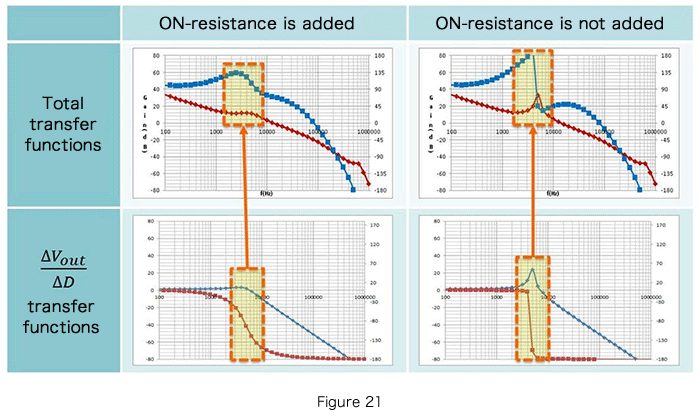Technical Information Site of Power Supply Design

2018.06.21 Transfer Function

# Effect of the ON-Resistance of a Switch on the Transfer Functions

DC/DC Converters: Sharing of Transfer Functions among Control Systems

The last two sections were devoted to Part 1 and Part 2 of "Examples of Transfer Function Derivation for Step-Up/Step-Down Converters". This time, we will study the "Effect of the ON-Resistance of a Switch on the Transfer Functions".

In this study as well, we will take the same approach as used before. As before, the transfer functions to be derived are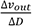and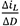, and once again, the transfer functions are derived in two steps.

Effect of the ON-Resistance of a Switch on the Transfer Functions

In transfer function derivations up till now, the effect of the ON-resistance of a switch (switching transistor) has not been considered. However, it is well known that in actuality a switch has an ON-resistance, and that the ON-resistance affects the actual operation. Here, we consider the effect of this parameter of the switch ON-resistance.

We start with the step-up/step-down converter for which ton≠ton’ in " Example of Transfer Function Derivation for a Step-Up/Step-Down Converter - 2 ", and use a similar procedure.

The circuit on the right is the simplified circuit for the step-up/step-down converter described the last time, with the ON-resistance for the MOSFET that is the switch indicated by RONp and RONn.

●Step 1: Consider the stable states of the system

① The coil current does not change over
one period
② The capacitor charge amount does
not change over one period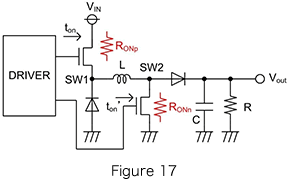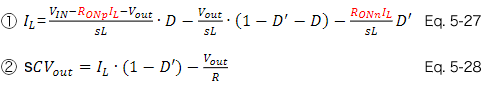Terms (in red) relating to the ON-resistance are added to the equation.

●Step 2: Determine change amounts for an external disturbance, and describe the transfer functions

A calculation example from equations 5-27 and 5-28 above is shown below.
Upon substituting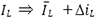,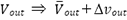,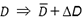in equations 5-27 and 5-28, the following is obtained.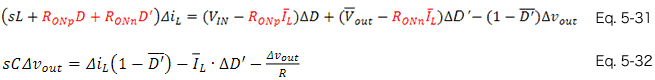Taking equations 5-31 and 5-32 as a system of simultaneous equations and determiningand, we obtain the following.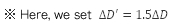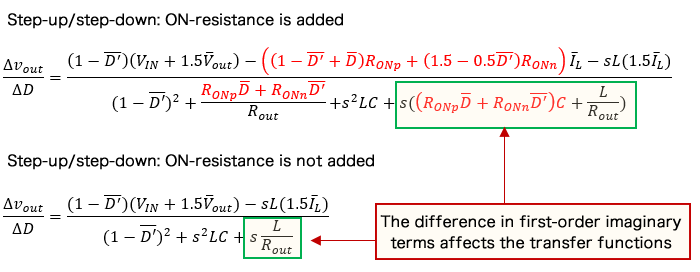As can be seen from the results, the first-order imaginary terms differ considerably. This is as was explained in the chapter "What are Transfer Functions"; here, we present only the characteristic results in the graphs that follow.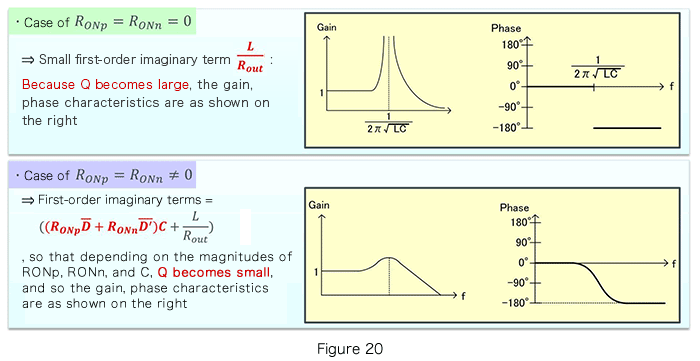Finally, the total transfer functions with and without the ON-resistance added are summarized below.